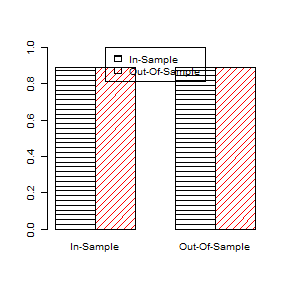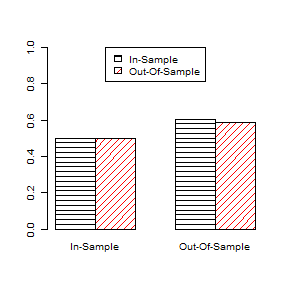# DC: Loan default prediction

library(ldt)
library(kableExtra)

It is recommended to read the following vignettes first:

## Introduction

In ldt, we automatically explain or automatically predict one or more than one random variable. In this vignette, we design a model set for predicting loan default. Of course, we focus on comparing the performance of logit and probit models. We use AUC as a performance measure:

measureOptions <- GetMeasureOptions(
typesIn = c("aucIn", "frequency"),
typesOut = c("aucOut", "frequency")
)

Note that, we calculate both in-sample and out-of-sample AUC. Also, we calculate the error ratio by using the following frequency cost matrix:

$\begin{equation} \label{eq:error-ratio} \begin{bmatrix} 0.5 & 1 & 1\\ 1.0 & 0 & 0 \end{bmatrix} \end{equation}$

which is:

frequencyCost <- matrix(c(0.5, 1, 1, 0, 1, 0), 2, 3)

Note that this is not a favorable cost matrix in an actual application. One might want to define more thresholds or increase the costs in the third column.

What is a frequency cost matrix in ldt? The general form of a frequency cost matrix in binary case is: $\begin{equation} \begin{bmatrix} t_1 & c_{11} & c_{12}\\ t_2 & c_{21} & c_{22}\\ \vdots & \vdots&\vdots\\ t_n & c_{n1} & c_{n2}\\ \end{bmatrix} \end{equation}$ In this presentation, $$t_i$$ for $$i=1,\ldots,n$$ is the threshold for the predicted probability. If the actual value is negative (i.e., $$y_i=0$$), the cost is determined by the first column. Otherwise (i.e., $$y_i=1$$), the cost is determined by the third column.

## Data

In this vignette, we use Berka and Sochorova (1993) data-set and ldt::Data_BerkaLoan() function to get a sample for the dependent variable and the potential predictors (or, features):

data <- Data_BerkaLoan(positive = c("B", "D"), negative = c("A", "C"))
#data <- Data_BerkaLoan(positive = c("B"), negative = c("A"))

The data set has a loan table with 682 observations, each labeled as one of the following:

• A: finished (29.8%);
• B: finished with default (vig_data$berka$B_finished%);
• C: running (59.1%); and
• D: running with default (6.6%).

Numbers in the parenthesis show the percentage of data in each class. Each loan observation has an account identification that can provide other types of information from other tables, such as the characteristics of the account of the loan and its transactions. Furthermore, each account has a district identification that can provide information about the demographic characteristics of the location of its branch. The combined table has features (including the label) and observations.

For this example, both finished and running (without default) classes are considered to be negative and both finished and running with default classes to be positive observations. Note that the observations labeled running might introduce measurement errors. Without them, the length of the table is smaller. If you do not want to use this part of the data, uncomment the code in the previous chunk.

The dependent and potential exogenous variables are:

y <- data[, c("label"), drop = FALSE]
x <- data[, 4:ncol(data)]

Note that the first 2 columns of data are id and status.

## Estimation

We are not able to load the data in this vignette, because it needs an external data set and this is not available in this package. But, a part of the data set is saved in the ldt package, and we load:

x = as.matrix(ldt::vig_data$berka$x)
y = as.matrix(ldt::vig_data$berka$y)

If you have downloaded the data set files, do not run this code. Since only 22.28739% of the observations are positive, we define and use the following weight vector to balance the data:

weight <- as.matrix((y == 1) * (nrow(y) / sum(y == 1)) + (y == 0))

There are 10 potential predictors and the size of the potential predicting models is relatively large. We follow a step-wise search approach by defining the following two arguments for the DcSearch_s() function:

xSizes <- list(as.integer(c(1, 2)), as.integer(c(3)))
xCounts <- c(NA, 4)

We also need a seed for the RNG and some other options to define the out-of-sample prediction:

measureOptions$seed <- 340 measureOptions$simFixSize <- 10
measureOptions\$trainRatio <- 0.75

Note that the out-of-sample simulation depends on random sampling. Finally, we start the search function:

berka_res <- list(
logit = DcSearch_s(
x = x, y = y, w = weight, costMatrices = list(frequencyCost),
xSizes = xSizes, counts = xCounts,
searchLogit = TRUE, searchProbit = FALSE,
searchItems = GetSearchItems(bestK = 20, inclusion = TRUE),
measureOptions = measureOptions,
searchOptions = GetSearchOptions(printMsg = FALSE),
savePre = NULL
),
probit = DcSearch_s(
x = x, y = y, w = weight, costMatrices = list(frequencyCost),
xSizes = xSizes, counts = xCounts,
searchLogit = FALSE, searchProbit = TRUE,
searchItems = GetSearchItems(bestK = 20, inclusion = TRUE),
measureOptions = measureOptions,
searchOptions = GetSearchOptions(printMsg = FALSE),
savePre = NULL
)
)

Since we want to compare the performance of logit and probit models, we run two discrete choice searches. All options are similar, but one is with searchLogit = TRUE and the other is with searchLogit = FALSE and searchProbit = TRUE. The results are reported in the following plot:Comparing the performance of best logit model and best probit model (cost-matrix and AUC)